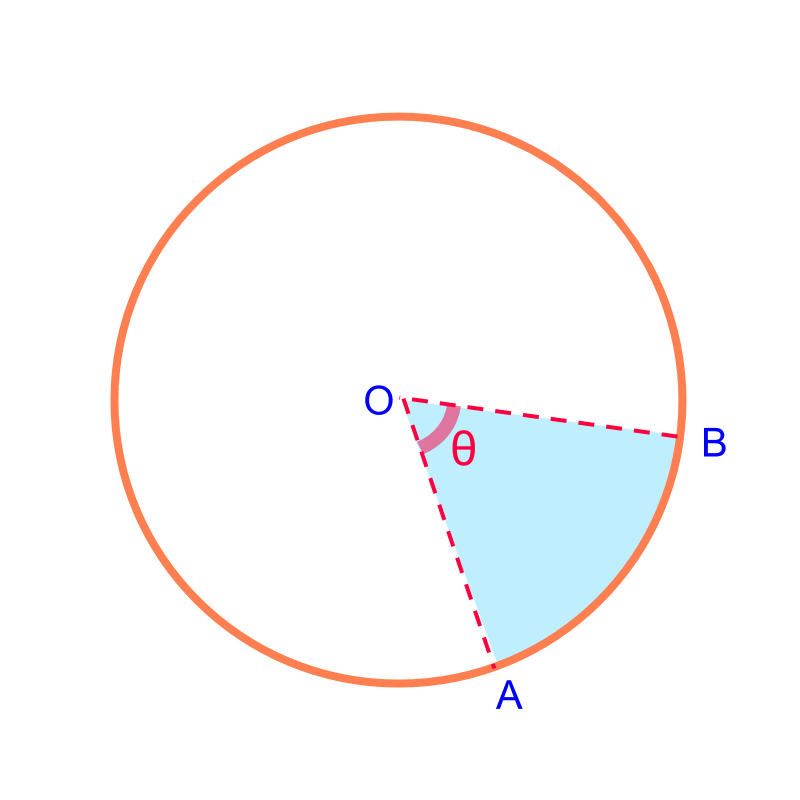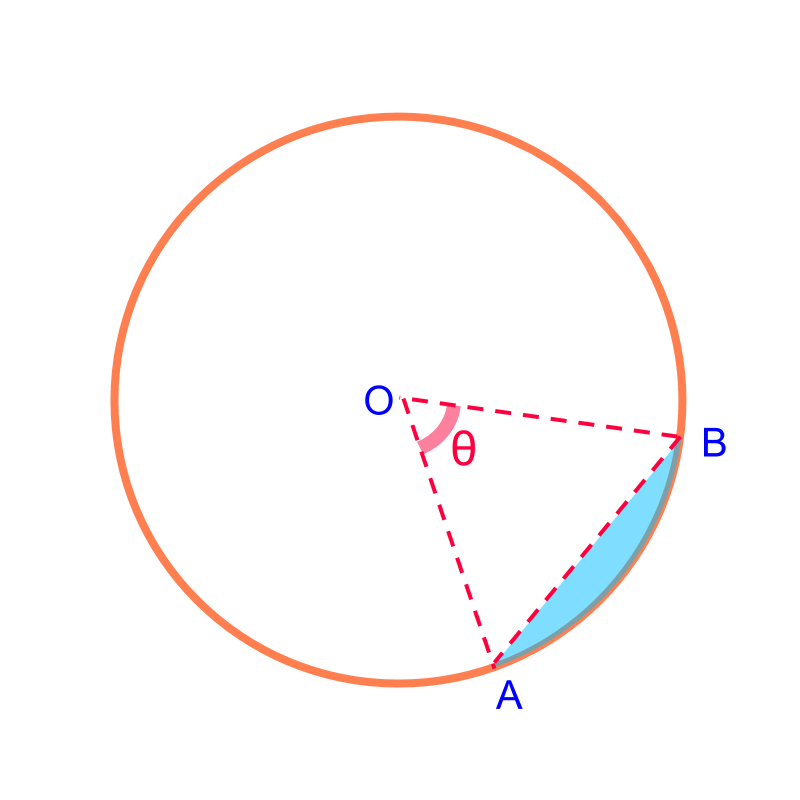maths > mensuration-high

Area of Parts of Circle : Sector and Segment

what you'll learn...

OverviewArc Length and Area of a Sector: The arc-length and area are proportion to the angle subtended by the sector.

Arc length of a sector of ${\theta }^{\circ }$${\theta}^{\circ}$ angle $=2\pi r×\frac{\theta }{360}$$= 2 \pi r \times \frac{\theta}{360}$

Area of a sector of ${\theta }^{\circ }$${\theta}^{\circ}$ angle $=\pi {r}^{2}×\frac{\theta }{360}$$= \pi {r}^{2} \times \frac{\theta}{360}$Area of a Segment: is the difference between area of sector and the triangle.
Area of a Segment of ${\theta }^{\circ }$${\theta}^{\circ}$ angle = area of the corresponding sector $-$$-$ the triangle

recap circle

The perimeter and area of a circle of radius $r$$r$ is "$2\pi r$$2 \pi r$ and $\pi {r}^{2}$$\pi {r}^{2}$"

The angle subtended by a circle on the center is "${360}^{\circ }$${360}^{\circ}$"

sectorConsider the sector of a circle shown in the figure. The parameters required to define the sector are "the radius and the angle subtended by the sector"

We learned that

Perimeter of a circle is $2\pi r$$2 \pi r$

Area of a circle is $\pi {r}^{2}$$\pi {r}^{2}$

Angle subtended by the entire circle is ${360}^{\circ }$${360}^{\circ}$

By the symmetry in the circle, it is found that the sector of angle $\theta$$\theta$ has

Arc length of a sector of $\theta$$\theta$ is $2\pi r×\frac{\theta }{360}$$2 \pi r \times \frac{\theta}{360}$. That is, the ratio of angle of the sector to full-angle is the ratio of perimeter of the sector to that of the full-circle.

Area of a sector of $\theta$$\theta$ is $\pi {r}^{2}\frac{\theta }{360}$$\pi {r}^{2} \frac{\theta}{360}$. That is, the ratio of angle of the sector to full-angle is the ratio of area of the sector to that of the full-circle.

measures of sectorConsider the sector given in figure. The arc length of minor arc $AB$$A B$ is "$2\pi r×\frac{\theta }{360}$$2 \pi r \times \frac{\theta}{360}$"

The perimeter of the shaded sector $OAB$$O A B$ is curve plus two radius length = $2\pi r×\frac{\theta }{360}+2r$$2 \pi r \times \frac{\theta}{360} + 2 r$

The area of the shaded sector $OAB$$O A B$ is "$\pi {r}^{2}×\frac{\theta }{360}$$\pi {r}^{2} \times \frac{\theta}{360}$".The area of the shaded segment is "Area of triangle $OAB$$O A B$ is subtracted from area of sector $OAB$$O A B$"

What is the area of a sector of ${180}^{\circ }$${180}^{\circ}$ and segment of ${180}^{\circ }$${180}^{\circ}$ in a circle?

The answer is "area of sector equals the area of segment $=\pi r$$= \pi r$"

summary

Arc Length and Area of a Sector:The arc-length and area are proportion to the angle subtended by the sector.

Arc length of a sector of ${\theta }^{\circ }$${\theta}^{\circ}$ angle $=2\pi r×\frac{\theta }{360}$$= 2 \pi r \times \frac{\theta}{360}$

Area of a sector of ${\theta }^{\circ }$${\theta}^{\circ}$ angle $=\pi {r}^{2}×\frac{\theta }{360}$$= \pi {r}^{2} \times \frac{\theta}{360}$

Area of a Segment: is the difference between area of sector and the triangle.Area of a Segment of ${\theta }^{\circ }$${\theta}^{\circ}$ angle = area of the corresponding sector $-$$-$ the triangle

Outline